211209 Epaper

 A Section B SectionC Section D Section211208 Epaper

 A Section B SectionC Section D Section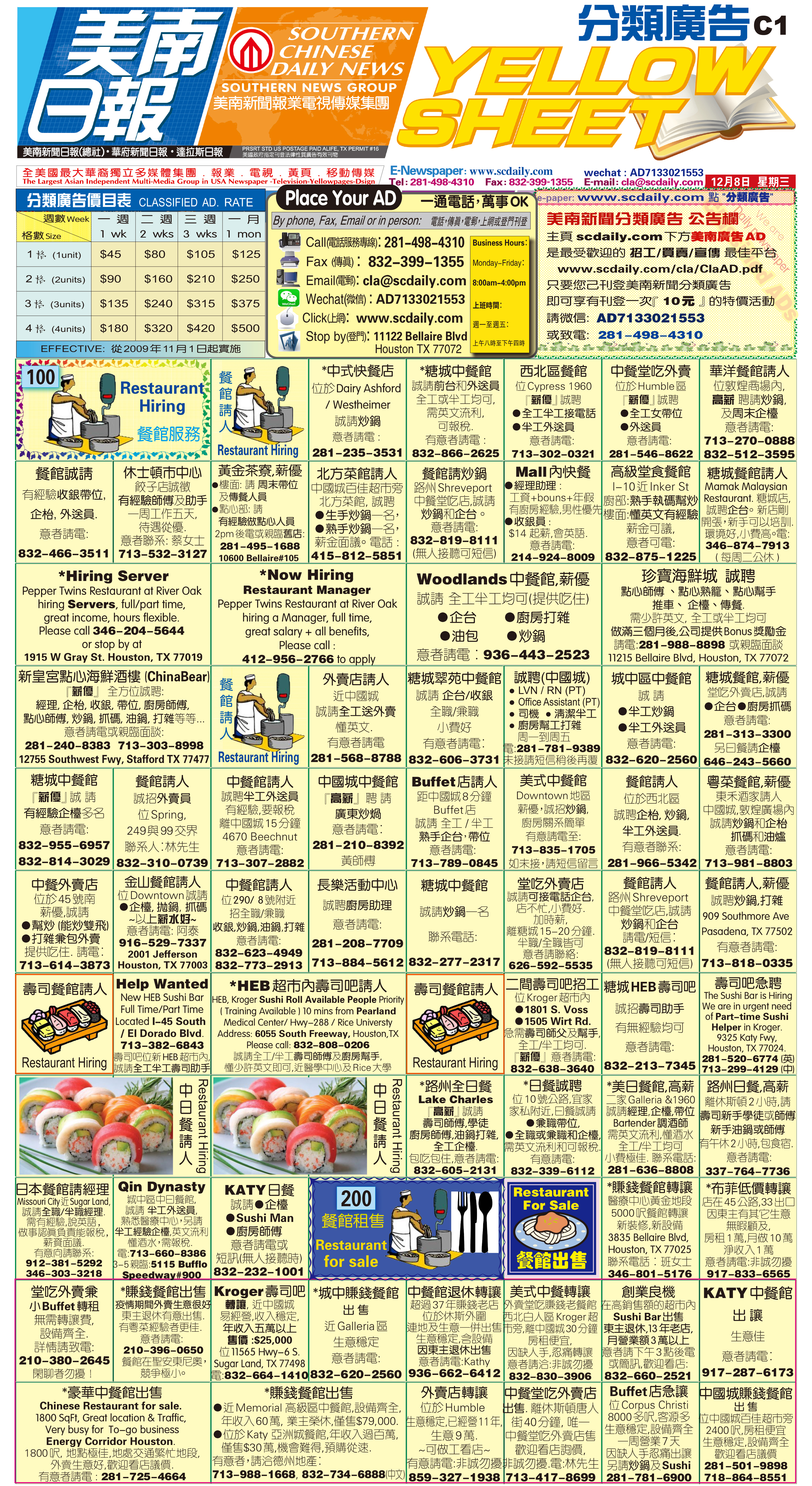211207 Epaper

 A Section B SectionC Section D Section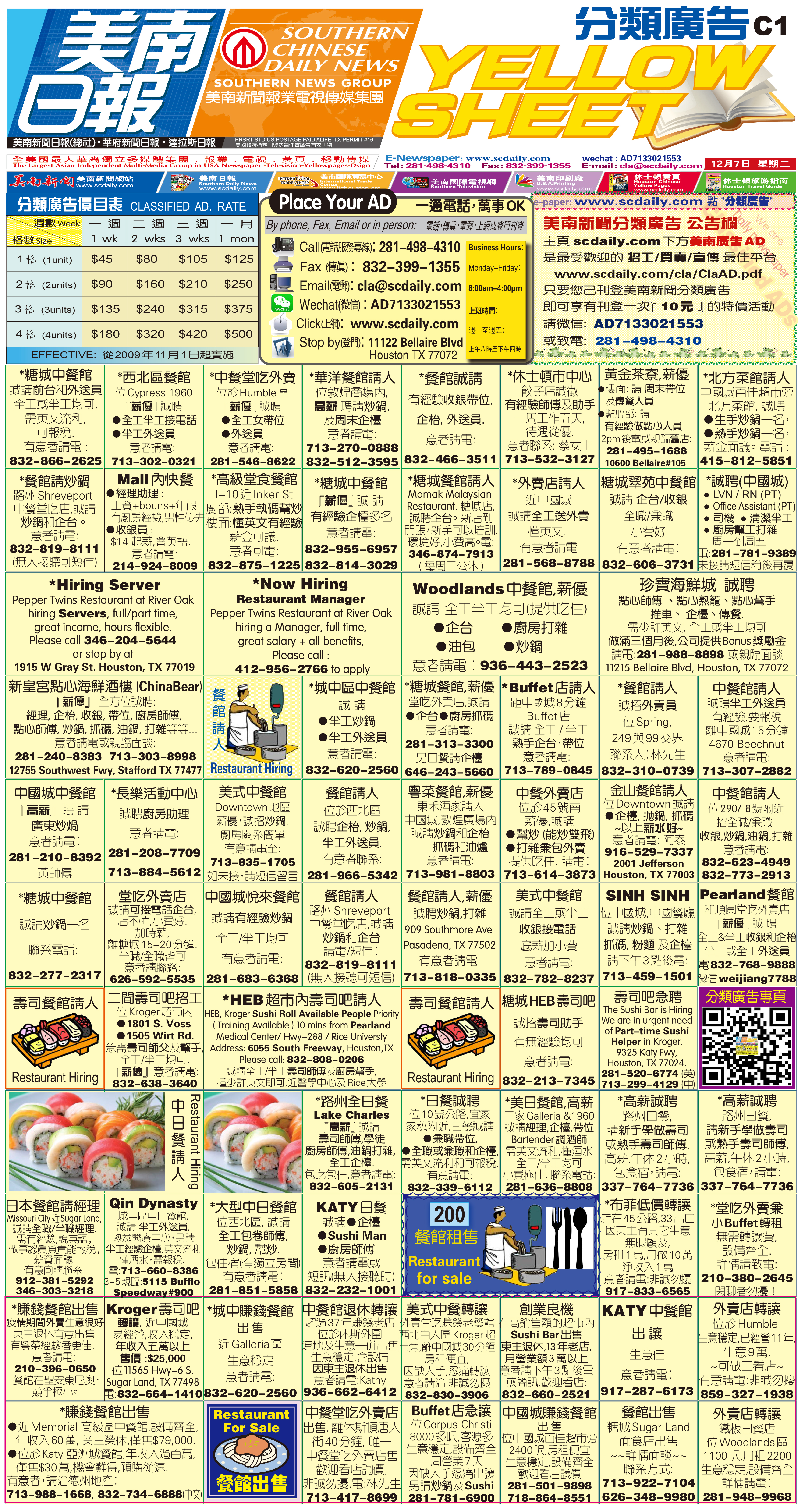211206 Epaper

 A Section B SectionC Section D Section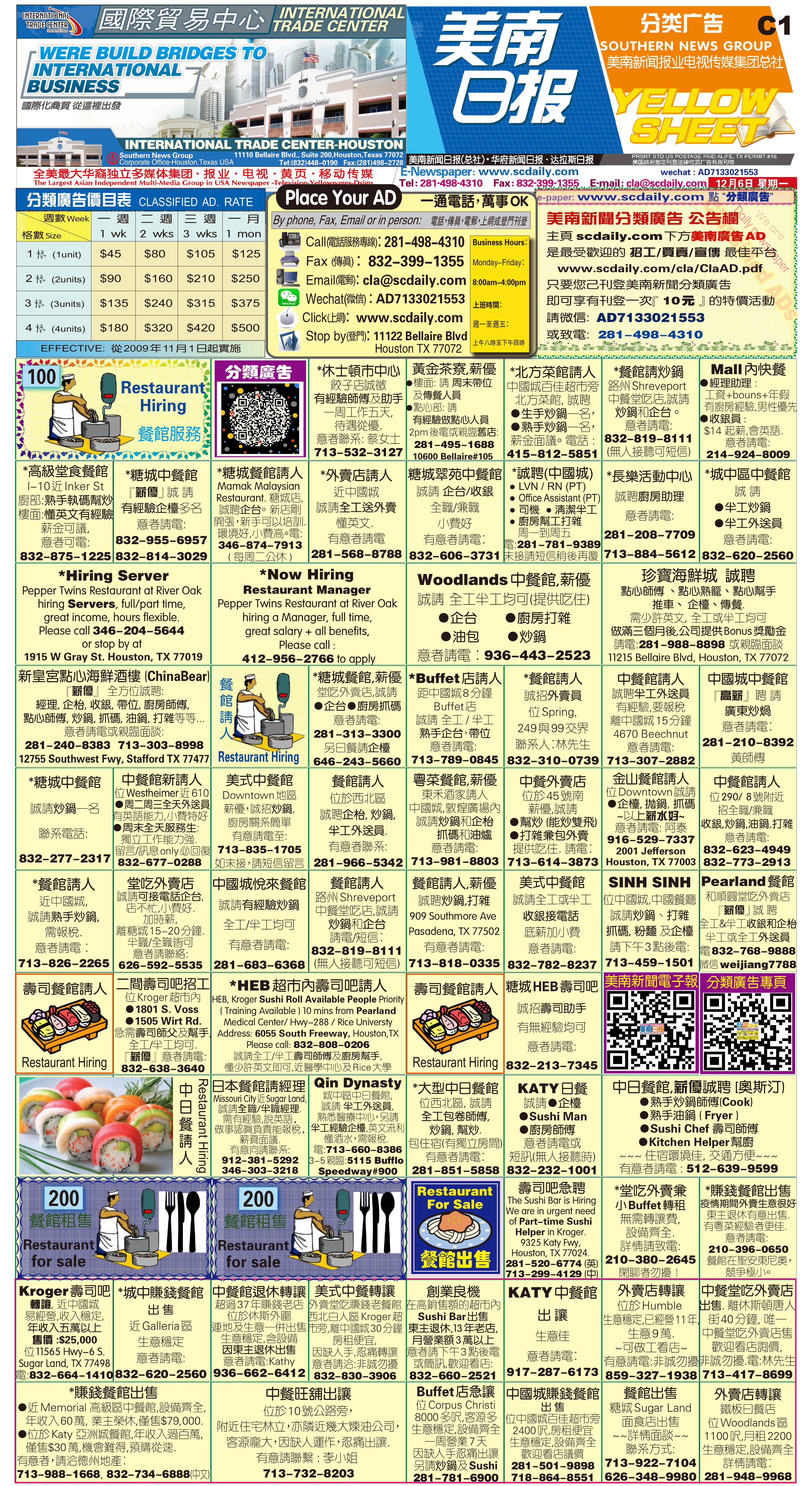211205 Epaper

 A Section B SectionC Section D Section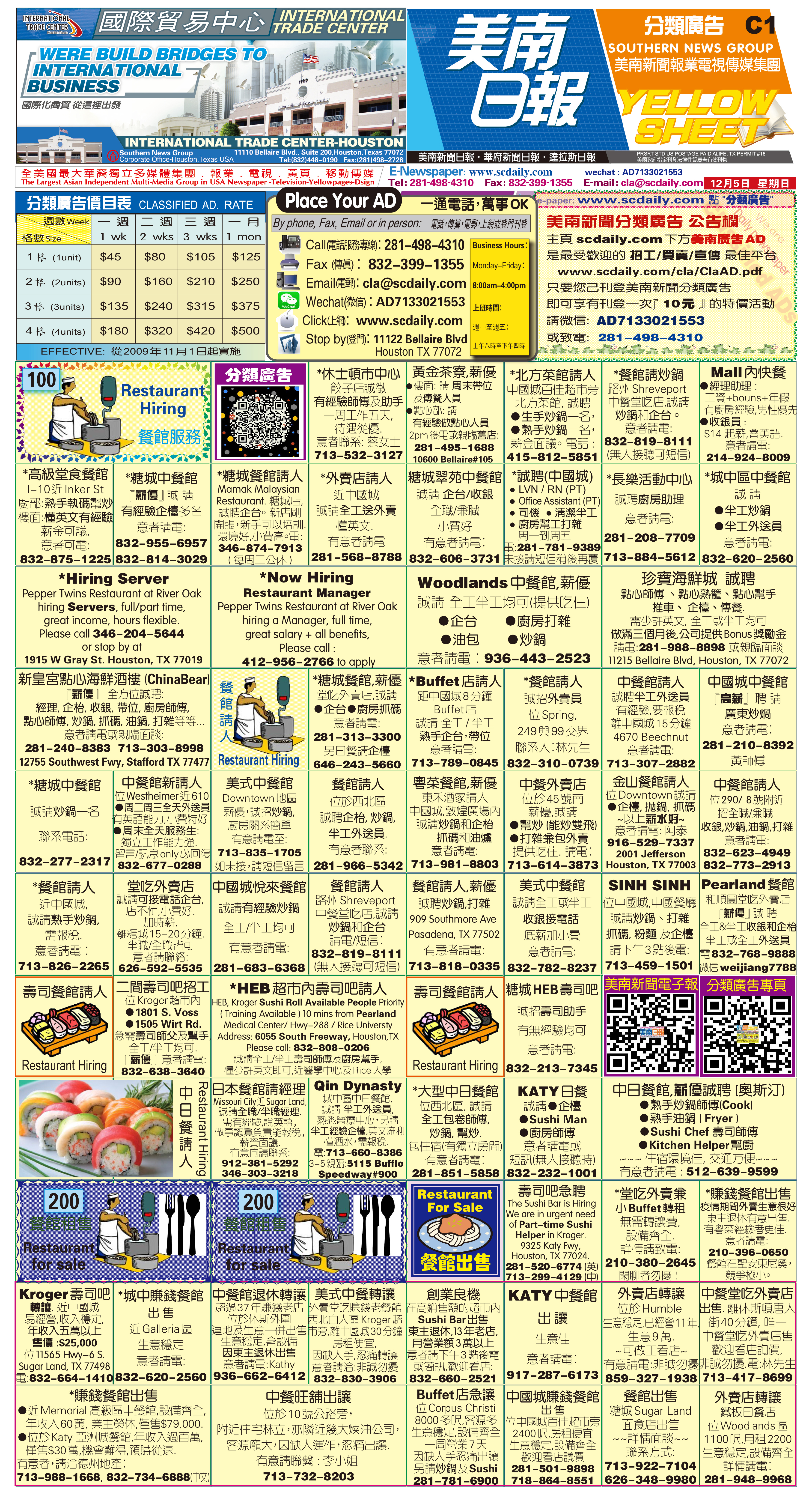211204 Epaper

 A Section B SectionC Section D Section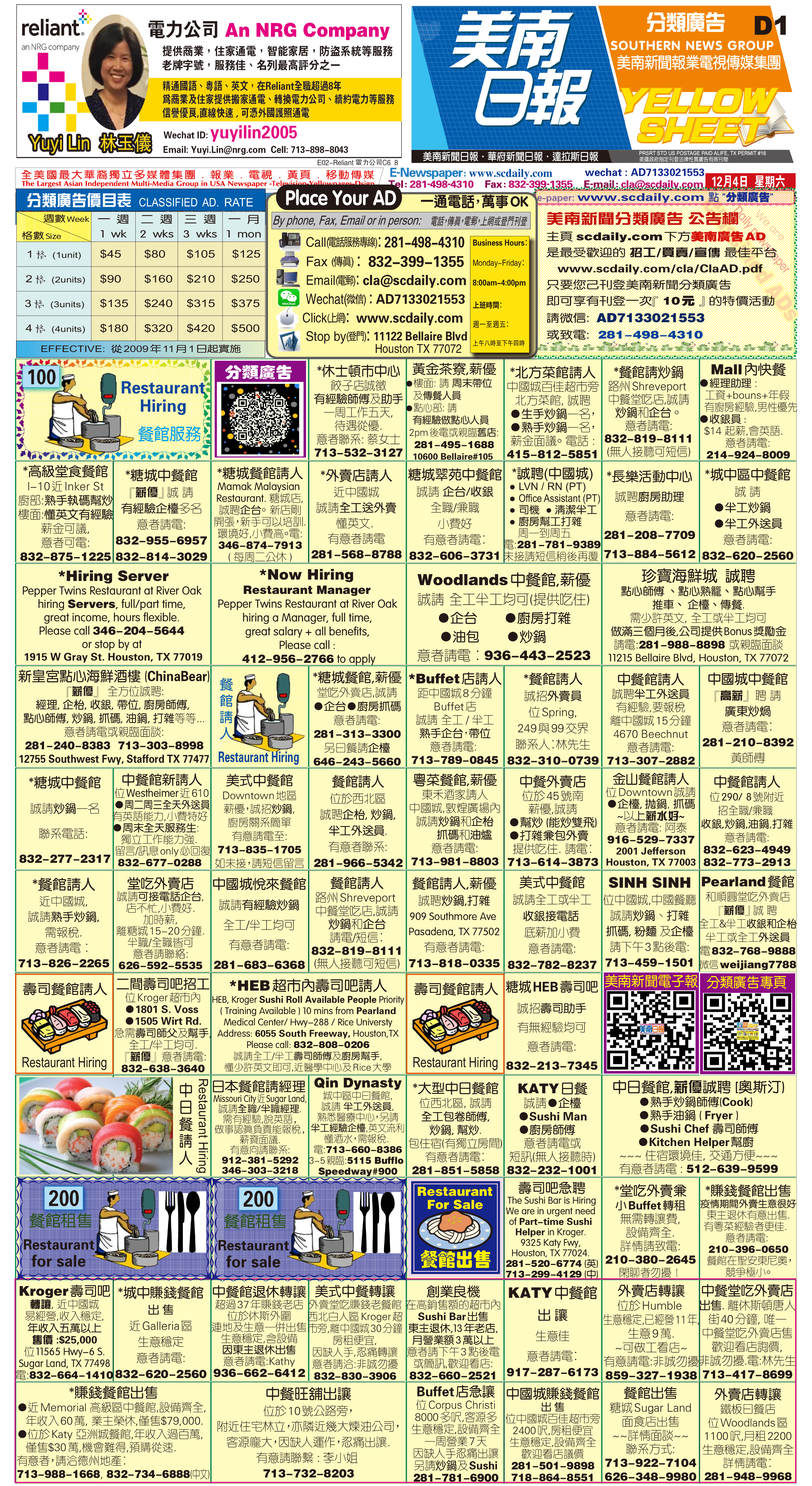211203 Epaper

 A Section B Section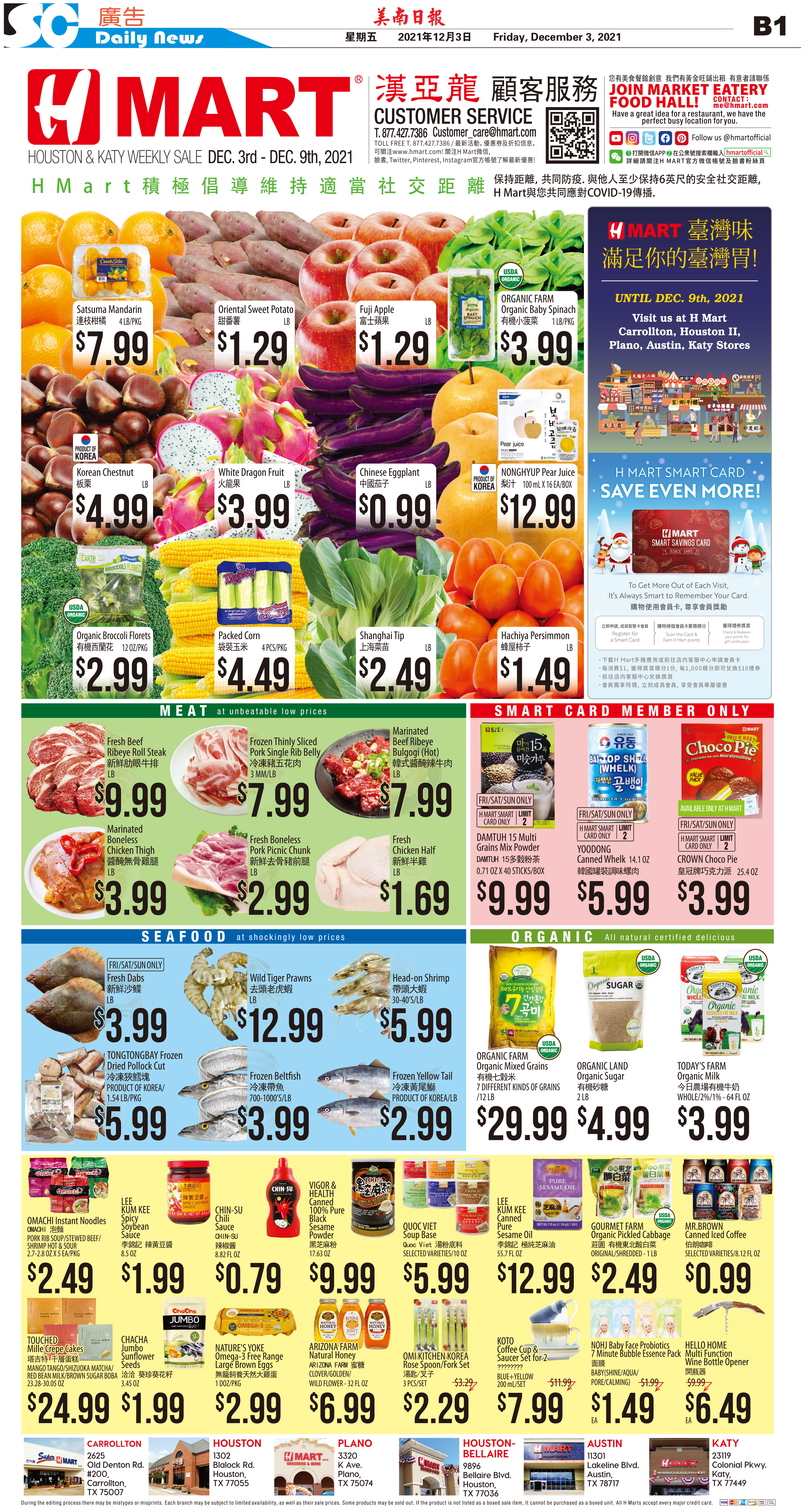C Section D Section211202 Epaper

 A Section B SectionC Section D Section211130 Epaper

 A Section B SectionC Section D Section211201 Epaper

 A Section B SectionC Section D Section211129 Epaper

 A Section B SectionC Section D Section